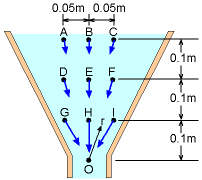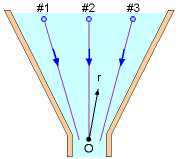Ch 3. Fluid Kinematics Multimedia Engineering Fluids FlowDescriptions Steady &Unsteady Streamlines,Streaklines Velocity &Acceleration IrrotationalFlow
 Chapter 1. Basics 2. Fluid Statics 3. Kinematics 4. Laws (Integral) 5. Laws (Diff.) 6. Modeling/Similitude 7. Inviscid 8. Viscous 9. External Flow 10. Open-Channel Appendix Basic Math Units Basic Equations Water/Air Tables Sections Search eBooks Dynamics Fluids Math Mechanics Statics Thermodynamics Author(s): Chean Chin Ngo Kurt Gramoll ©Kurt GramollFLUID MECHANICS - CASE STUDY

IntroductionSchematic of Experiment Set #1Schematic of Experiment Set #2

In a laboratory, two sets of experiments are conducted to measure the steady velocity in a funnel. The first set of the experimental data is collected at fixed locations, as shown in the figure. The measured velocities are:

 Sensor Location A B C Velocity (V), cm/s 5.2 5.6 5.5 Sensor Location D E F Velocity (V), cm/s 11.5 12.4 11.7 Sensor Location G H I Velocity (V), cm/s 40.0 50.5 39.5

The second set of the experimental data is obtained by following three different fluid particles. As the fluid particle travels along its path, the velocity and its location (with respect to the origin, point O) are measured randomly:

 Fluid Particle #1 Location (r), m 0.25 0.18 0.07 Velocity (V), cm/s 8.1 15.5 101.8 Fluid Particle #2 Location (r), m 0.30 0.25 0.19 Velocity (V), cm/s 5.7 7.8 14.0 Fluid Particle #3 Location (r), m 0.21 0.17 0.10 Velocity (V), cm/s 11.0 16.9 50.0

Assume that the velocity is inversely proportional to the square of the location r (see figures). Students are asked to find a correlation to describe the velocity field.

Questions

Identify which viewpoint (Eulerian or Lagrangian) is used for the experiment. Also, find a correlation for the velocity.

Approach

• Determine the correlation based on the average value of the collected experimental data.
• The flow is steady. Hence, it is not a function of time.
• The flow is assumed to be a function of the location r only.

Practice Homework and Test problems now available in the 'Eng Fluids' mobile app
Includes over 250 problems with complete detailed solutions.
Available now at the Google Play Store and Apple App Store.